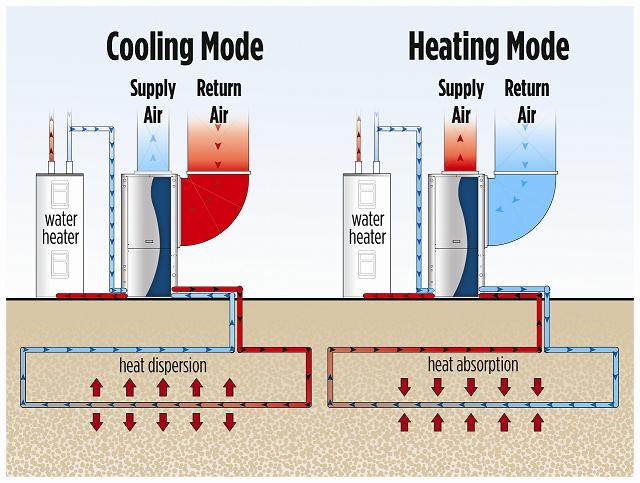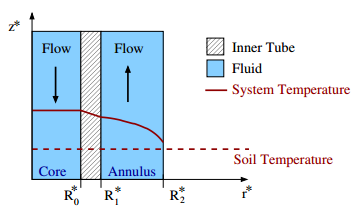# Spencer Frei

Department of Statistics
University of California, Los Angeles

Email: spencerfrei@ucla.edu

I am a PhD candidate in the Statistics department at UCLA and a member of the Statistical Machine Learning Lab led by Quanquan Gu. I'm interested in optimization and generalization in deep learning and other problems in statistical learning. I also develop the deep learning algorithms that power the Chatterbaby app.

### Research Interests

* Theory of deep learning: optimization, generalization, representation learning, etc.
* Statistical learning theory
* Non-convex optimization
* Applications of deep learning: natural language understanding, audio analysis, etc.

### Recent news

2020
* I will be attending the IDEAL Special Quarter on the Theory of Deep Learning hosted by TTIC/Northwestern for the fall quarter.
* I'm reviewing for AISTATS 2021.
* Updated the arXiv version of my recent paper on agnostic learning of a single neuron with improved bounds.
* I've been awarded a Dissertation Year Fellowship by UCLA's Graduate Division.
* New paper on agnostic PAC learning of a single neuron using gradient descent is now on arXiv.
* New paper accepted at Brain Structure and Function from work with researchers at UCLA School of Medicine.
* I'll be (remotely) working at Amazon's Alexa AI group for the summer as a research intern, working on natural language understanding.
* I'm reviewing for NeurIPS 2020.
* I'm reviewing for ICML 2020.

2019
* My paper with Yuan Cao and Quanquan Gu, "Algorithm-dependent Generalization Bounds for Overparameterized Deep Residual Networks", was accepted at NeurIPS 2019 (arXiv version, NeurIPS version).

I am currently a PhD candidate in the Statistics department at UCLA and a member of the Statistical Machine Learning Lab.  I am supervised by Ying Nian Wu from the Department of Statistics and Quanquan Gu from the Department of Computer Science. I completed my masters in mathematics at the University of British Columbia, Vancouver, in May 2015.  I was a member of the Probability Group, and Ed Perkins was my supervisor.  Before that, I completed my undergraduate degree in mathematics at McGill University in 2013.

For 2020-2021, I have a UCLA Dissertation Year Fellowship and will not be teaching.

Past teaching positions:
Spring 2020: Stats 100C, Linear Models with Arash Amini.
Fall 2019: Stats 102C, Monte Carlo Methods with Qing Zhou.
Summer 2016, Session C: Stats 10, Intro Statistics with Juana Sanchez.
Summer 2016, Session A: Stats 10, Intro Statistics with Miles Chen.
Fall 2016: Stats 100A, Introduction to Probability Theory with Ying Nian Wu.
Winter 2016: Stats 100B, Introduction to Mathematical Statistics with Jessica Li.

Since Fall 2017, I have been working as a graduate student researcher (GSR) for Ariana Anderson at the Semel Institute for Neuroscience and Human Behavior. For the 2016-2017 school year, I worked as a GSR for Ariana Anderson and Monika Mellem.

#### Preprints

1. S. Frei, Y. Cao, and Q. Gu. Agnostic learning of a single neuron with gradient descent. Preprint, 2020.

#### Refereed Conference Publications

2. S. Frei, Y. Cao, and Q. Gu. Algorithm-dependent generalization bounds for overparameterized deep residual networks. Conference on Neural Information Processing Systems (NeurIPS), 2019.

#### Journal Publications

3. A.E. Anderson, M. Diaz-Santos, S. Frei et al. Hemodynamic latency is associated with reduced intelligence across the lifespan: an fMRI DCM study of aging, cerebrovascular integrity, and cognitive ability. Brain Structure and Function, 2020

4. S. Frei and E. Perkins. A lower bound for the critical probability in range-$R$ bond percolation on $\mathbb Z^d$ via SIR epidemic methods. Electronic Journal of Probability 21(56), 2016.

5. S. Frei, K. Lockwood, G. Stewart, J. Boyer, and B.S. Tilley. On thermal resistance in concentric residential geothermal heat exchangers. Journal of Engineering Mathematics 86(1), 2014.

Below is a list of some of the projects I have worked on.  Note that this page utilizes MathJax rendering of LaTeX code.

### SIR epidemics and range-R percolation on $$\mathbb{Z}^d$$

One can construct a discrete-time mathematical SIR (susceptible, infected, recovered) epidemic on a graph $$G = (V,E)$$ by partitioning the elements in $$V$$ into one of three sites: susceptible $$(x\in \xi_n)$$, infected $$(x\in \eta_n)$$, and recovered $$(x\in \rho_n)$$. We start from an initial configuration of susceptible and infected sites (say, $$\eta_0 = \{ 0\}$$, $$\xi_0 = V \setminus \eta_0$$, and $$\rho_0=\emptyset$$), and we consider a fixed parameter $$p\in (0,1)$$ that is the probability that any infected site $$x\in \eta_n$$ infects a neighboring susceptible site $$y\in \xi_n$$ (so that $$(x,y)\in E)$$. Infected sites remain infected for one unit of time, and then become recovered’, and may no longer infect any other sites (or become infected again); that is, $$x\in \eta_n$$ means $$x\in \rho_{n+1}$$. We then let the process run for times $$n=1, 2, \dots$$ and examine the behavior of the infected sites $$\eta_n$$.

There are two possible behaviors for the epidemic: either the epidemic dies out in finite time almost surely (i.e., $$\eta_n = \emptyset$$ eventually), or with positive probability the epidemic continues forever, so that at any given time $$m$$, there is at least one site $$x\in \eta_m$$. In the latter case, we say that infection occurs in the epidemic. Since each edge $$(x,y)$$ in the epidemic process is used’ precisely once—when either $$x$$ attempts to infect $$y$$ or vice-versa—there is a correspondence between infections occurring in a graph $$G$$ and percolation occurring in the same graph: an infection occurs in $$G$$ iff percolation occurs in $$G$$. Standard percolation theory shows that there exists a critical value $$p_c(G)$$ for the probability $$p$$ such that when $$p>p_c$$, infection occurs, and for $$p<p_c$$, the epidemic dies out almost surely.

I studied this process on the graph $$\mathbb Z^d$$, with an edge set $$E = E_R$$ parameterized by a quantity $$R$$, such that there are edges between two sites $$x,y\in \mathbb Z^d$$ iff $$|x-y|_{\infty} \leq R$$, where $$|\cdot|_\infty$$ denotes distance in the $$\ell^\infty$$ metric. As the graph is parameterized by $$R$$, the corresponding critical value $$p_c$$ is likewise paramaterized by $$R$$. In my masters thesis, I proved a lower bound on the critical value for percolation, as a function of $$R$$, in dimensions $$d=2$$ and $$d=3$$ by studying the corresponding SIR epidemic. A paper on this result, in collaboration with Ed Perkins, is available at the Electronic Journal of Probability.

### Mathematical model of geothermal heating systemsGeothermal heat pump systems (also known as ground-source heat pump systems, or GSHP) are an incredibly energy-efficient way to heat and cool a home.  Traditional heat pumps rely on a heat exchanger that exchanges heat with air, which functions as a heat source/sink.  Geothermal heat pump systems utilize the fact that just a few meters below the surface, the ground is nearly constant temperature year-round, and hence use the soil as a heat source/sink.  The heat exchanger takes the form of a liquid running through pipes that are burrowed underground.   As the temperature of the soil is near-constant year round, the heat pump is also able to function effectively year-round, as the liquid through the pipes deposits heat into the soil in the summer, and receives energy from the soil in the winter. The pipes may be situated in a number of networks, most of which are described at the Department of Energy website

The coefficient-of-performance, a common performance metric for heating systems that is defined as the energy output (in heat) of a system per energy input (in electricity to run the system), for GSHP is approximately 4-7, while traditional heat pump systems that utilize air as the heat exchange medium have coefficients of performance of approximately 2.  The main deterrent to widespread adoption of ground source heat-pump systems is the large initial investment cost (on the order of \$10,000s) which is recuperated in energy savings over approximately 10 years.  The initial investment is largely due to the drilling of deep (100-300 feet) boreholes that are approximately 1-2 feet in diameter that house the pipes that allow the water to exchange heat with the soil through the highly conductive material constituting the pipe walls.  And, although it is well-known that these systems are highly effective in certain geological/climatological conditions, there is little knowledge of the mathematics underpinning the process of heat exchange through the pipe walls.  It is particularly important to understand how deep one must drill the boreholes in order for the heat-pump system to function properly, as this is the main contribution to the installment cost.I worked with Burt Tilley, Kathryn Lockwood, Greg Stewart, and Justin Boyer to develop a mathematical model of the heat exchange occurring between the water in the pipes, the pipe walls, and the soil in a specific piping system known as the concentric vertical pipe.  In this pipe, water flows down from the home through an inner cylindrical cavity, and flows back up the pipe to the home through an outer cylindrical cavity.  The diagram to the left depicts the system in action during summer, as the water decreases in temperature as it exchanges heat from the soil.  The pipes are designed so that the inner pipe material in the region $$R_0^* < r^* < R_1^*$$ has low conductivity while the outer pipe material at $$r^* = R_2^*$$ is highly conductive.  Our model studied the heat exchange as a function of design parameters such as the spacing of the inner and outer pipes, the flow rate, and the length of the pipe.  We developed a characteristic length at which heat exchange occurs in the soil, and found that one could drill less deeply while still retaining the vast majority of heat exchange ability of the system.  These results were published in the Journal of Engineering Mathematics in 2014.